## 故事的起因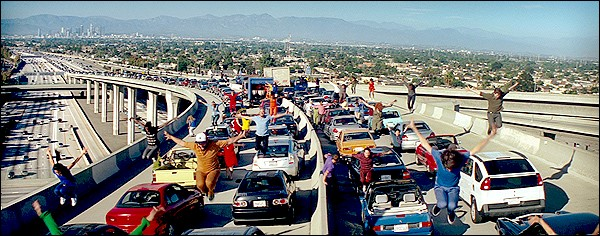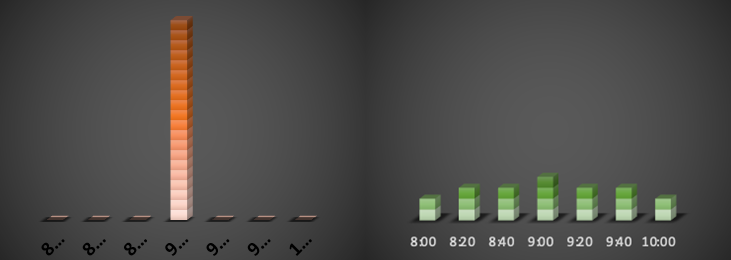## “奇怪”的用户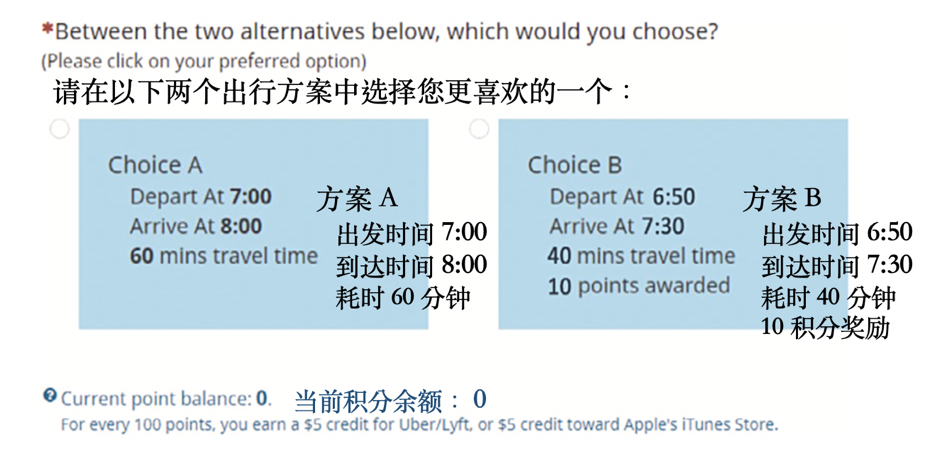$$Utility=\beta_0+\beta_1 x_1+\beta_2 x_2+\beta_3 x_3+\beta_4 x_4+\epsilon$$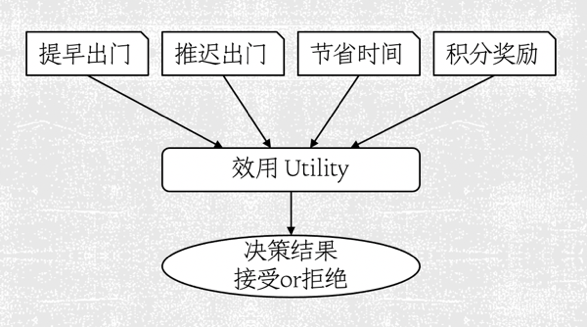## 一个新的解释

1. 系统给出的每一种出行方案，根据其具体内容（比如提前多早出门，节省多少时间），会对用户构成一个成本。而这个系统给出的出行方案离用户原有的方案差距越大，用户接受这个方案的成本就越高。
2. 只有当积分奖励大于这个用户的成本的情况下，用户才有可能接受这个系统推荐的出行方案。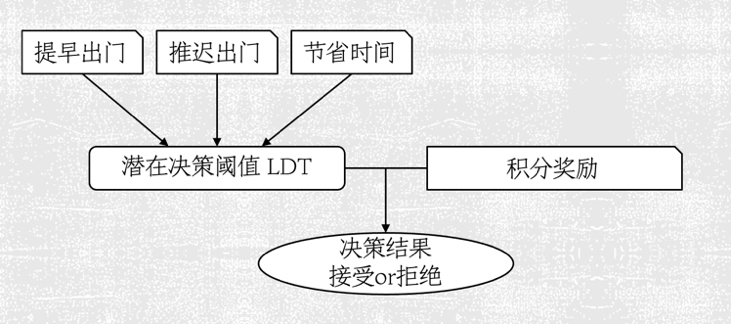## 潜在决策阈值模型 [LDT model]

$$y(r-f(x_1,x_2,x_3))\geq 0$$

\begin{aligned} \min_{\alpha_{0}, \alpha, \xi} \qquad &\frac{1}{2} \boldsymbol{\alpha}^{\top} \boldsymbol{\alpha}+C \sum_{t} \xi_{t} \\ \text { s.t. } & y_{t}\left(r_{t}-\alpha_{0}-\boldsymbol{\alpha}^{\top} \boldsymbol{x}_{t}\right) \geq 1-\xi_{t}, \text { for all } t, \\ & \xi_{t} \geq 0, \text { for all } t, \end{aligned}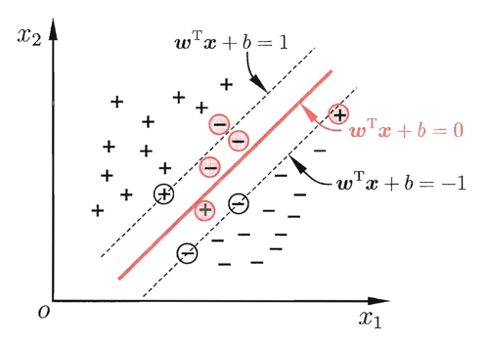## 解释 LDT

RUM LDT
$\beta_3$，节省时间 21.25% 13.15%
$\beta_4$，积分奖励 38.84% 0

## 参考文献

 Feng, J., Huang, S., & Chen, C. (2020). Modeling user interaction with app-based reward system: A graphical model approach integrated with max-margin learning. Transportation Research Part C: Emerging Technologies, 120, 102814.

 Zhu, X., Wang, F., Chen, C., & Reed, D. D. (2020). Personalized incentives for promoting sustainable travel behaviors. Transportation Research Part C: Emerging Technologies, 113, 314-331.